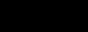Learn Anytime

 Home English Maths Science History Geography French Spanish Technology Art P.S.H.E R.E I.C.T Music Games Downloads
 Drawing Lines Demonstration Activities Depth Space a short animation explaining the concept Edge | Outline a short animation explaining the concept Geometric | Organic Shape a short animation explaining the concept Line a short animation explaining the concept Linear | Aerial Space a short animation explaining the concept Positive |  Negative Space a short animation explaining the concept Symmetrical | Asymmetrical Balance a short animation explaining the concept Visual Movement | Rhythm a short animation explaining the concept Visual Repetition | Pattern a short animation explaining the concept Practice Activities Draw lines which depict feelings some example words are given Draw two cities one where all the lines are straight and the other all curvy Extension Activities Depth Space an activity where learners practise skills shown in demonstration Edge | Outline an activity where learners practise skills shown in demonstration Geometric | Organic Shape an activity where learners practise skills shown in demonstration Line | an activity where learners practise skills shown in demonstration Linear | Aerial Space an activity where learners practise skills shown in demonstration Positive | Negative Space an activity where learners practise skills shown in demonstration Symmetrical | Asymmetrical Balance an activity where learners practise skills shown in demonstration Visual Movement | Rhythm an activity where learners practise skills shown in demonstration Visual Repetition | Pattern an activity where learners practise skills shown in demonstration Testing Activities Depth Space an example to test understanding of the concept Edge and Outline an example to test understanding of the concept Geometric | Organic Shape  an example to test understanding of the concept Line | an example to test understanding of the concept Linear | Aerial Space an example to test understanding of the concept Positive | Negative Space an example to test understanding of the concept Symmetrical | Asymmetric Balance an example to test understanding of the concept Visual Movement | Rhythm an example to test understanding of the concept Visual Repetition | Pattern an example to test understanding of the concept